• 日志
• 用户
• 版块

« 返回列表

# 主题：[影音分享]方文山第五届西塘汉服文化周圆满落幕wuli是亲故呀206

96

207

2016-04-25

# 方文山第五届西塘汉服文化周圆满落幕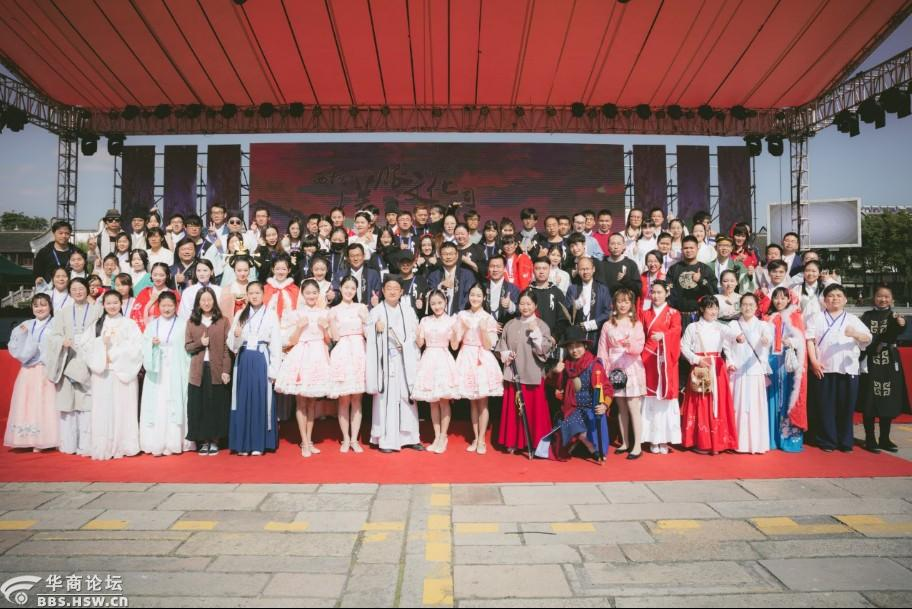[size=;12]2017年10月[size=;12]31[size=;12]日，第五届西塘汉服文化周活动[size=;12][size=;12]浙江嘉善县西塘古镇[size=;12]顺利拉上帷幕[size=;12]。自2013年方文山先生发起汉服文化周活动[size=;12]到现在，[size=;12]转眼间，西塘汉服文化周已经走[size=;12]到了[size=;12]第五年[size=;12][size=;12]不负众望，时至今日[size=;12]汉服文化周已经发展成为国内首屈一指的汉服文化活动盛事[size=;12][size=;12]同时也成为[size=;12]西塘[size=;12]古镇[size=;12]旅游文化[size=;12]名片。今年依旧有众多[size=;12]国内外中华传统文化爱好者相聚西塘[size=;12][size=;12]据不完全统计，活动期间日均人流量2万多人，商家100余家，社团300余家，这是广大商家、同袍同好以及传统文化爱好者对我们活动的肯定，也成为我们继续弘扬中华优秀传统文化的责任与动力[size=;12][size=;12]
[size=;12]回顾[size=;12]本届汉服文化周[size=;12][size=;12]诸多活动内容丰富，寓意深刻。除了[size=;12]朝代嘉年华、汉服好声音、[size=;12]汉服相亲大会、[size=;12]水上传统婚礼、国学好好玩、草船借箭等历届经典活动[size=;12]之外[size=;12]，更增添了霹雳之夜、小童星汉服T台秀、铠甲展、中华武备展演、“西塘杯”古诗词大赛等新元素和精彩内容[size=;12][size=;12]在开幕式上方文山先生和各位领导致欢迎辞为汉服文化周助力、演员徐娇领衔“汉服美少女”方阵惊艳亮相朝代嘉年华仍旧历历在目，此番盛况，让[size=;12]我们有理由相信[size=;12][size=;12]这不仅仅是一场汉服盛会[size=;12][size=;12]更是一场传统文化庆典，是传承优秀民族文化的[size=;12]盛事，是对中国几千年历史文明的回顾[size=;12][size=;12]更是[size=;12]对中华传统文化[size=;12]美好[size=;12]未来的展望[size=;12]......[size=;12]
[size=;12]

[size=;9]

[size=;12]今年恰逢汉服文化周5周年、西塘古镇旅游[size=;12]发展[size=;12]20周年，2017年西塘古镇旅游景区更是被国家旅游局授予国家AAAAA级旅游景区牌匾。西塘与汉服文化周携手走过五年，二者早已密不可分。因此，主办方在本届汉服文化周代入了[size=;12][size=;12]“520”概念，不仅表现了对汉服及中国传统文化的热爱和倡导，也承载着对千年历史古镇的深情。[size=;12]
[size=;12]为了更好地保护和传承西塘古镇悠久的历史文化及人文风情，嘉善县人民政府开展了关于“善文化”的系列活动。 第五届汉服文化周[size=;12]开幕式当天也[size=;12]进行[size=;12][size=;12]“满城荡漾善文化”的主题演讲，[size=;12]旨在[size=;12]进一步加强对“满城颂善”、“满城论善”、“满城行善”的社会风气的培育，[size=;12]鼓励广大群众参与到“善文化”活动中来，在全社会营造崇德向善的良好氛围，让“善文化”成为嘉善人的精神归宿。[size=;12]
[size=;12]

[size=;12]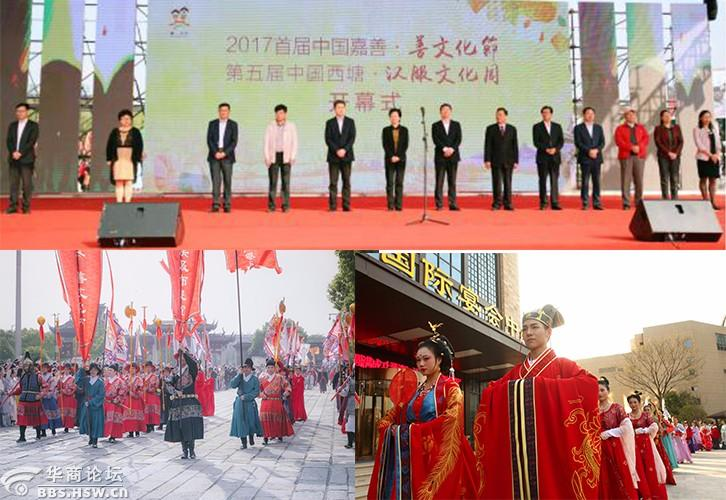[size=;12]2017第五届中国西塘汉服文化周于[size=;12][size=;12]上午9[size=;12][size=;12]30分[size=;12]在浙江嘉善西塘古镇正式开幕。[size=;12]第一环节是嘉善·善文化节开幕式[size=;12][size=;12]由嘉善县委常委、宣传部部长武耀云[size=;12]女士[size=;12]介绍到场领导嘉宾，浙江嘉兴市嘉善县委副书记徐鸣阳先生致欢迎词，随后嘉兴市委宣传部副部长姚伟先生和著名导演孙渝烽先生上台[size=;12]讲话[size=;12]，最后善文化节开幕式在《善城有爱》的悠扬歌声中圆满结束。[size=;12]紧接着，[size=;12]第五届西塘汉服文化周开幕式[size=;12]9:30[size=;12]正式开始，嘉善县副县长许春红、西塘镇党委书记王永根、嘉善县旅游局局长陆勇伟、嘉善西塘旅游休闲度假区管理委员会副主任陆丰莅临活动现场，汉服文化周活动发起人方文山先生到场见证，许春红先生与方文山老师共同完成启动仪式，随后方文山老师邀请徐娇小姐上台致辞。[size=;12]上午[size=;12]10[size=;12][size=;12]40[size=;12]分，[size=;12]现场领导和嘉宾共同释放气球热烈庆祝第五届西塘汉服文化周活动正式开幕。[size=;12]
[size=;12]本届汉服文化周开幕式依然是以朝代嘉年华作为开场，各个朝代方阵陆续登场。首仪仗参考《出警入跸图》，气势恢宏，尽显明代仪仗的威仪；方阵展演以短剧表演的形式，力求复原当时的历史人物风貌，描绘耳熟能详的典故，表现老百姓诉求的正能量；通过周、汉、晋、唐、宋、元、明、清各代传统服[size=;12]饰的展示，使参与者一览中国传统服饰的全貌、感受每个朝代其特有的人文特征及汉服一朝一代沿革的活力。其中的骑射、铠甲、婚礼方阵以及亲子方阵更是让人耳目一新，倍感惊艳。[size=;12]
[size=;12]西塘古镇所处的嘉善以培育和践行社会主义核心价值观为根本，全面打造“善文化”升级版。在“善文化节”活动开幕式上，嘉善县委与嘉善人民政府借助现有文化产业平台的招商资源，联合上海文创资源联盟、浙江省文化产业促进会等举办全县文化产业招商推介活动。在10月-12月期间，第七届道德模范评选表彰活动颁奖、“满城荡漾善文化”排舞大赛、了凡善学思想论坛、“善文化”主题青年辩论赛、“日行一善久成林”慈善活动等“善文化节”系列活动将有序展开。嘉善县人民政府响应国家复兴华夏优秀文化的号召，积极传播民族优秀正能量品德，是对“向善、学善、扬善”的善文化的深刻践行，此举[size=;12]可谓[size=;12]意义非凡。[size=;12]
[size=;12]西塘古镇有着江南的婉约气质，汉服文化的历史传承，更有善文化的精神支撑[size=;12][size=;12]今年的“嘉善•善文化节”及西塘汉服文化周[size=;12]得以[size=;12]顺利启航！[size=;12]
[size=;12][size=;12][size=;12][size=;12]活动除了往年的朝代嘉年华、汉服好声音、水上T台秀、汉服之夜、水上传统婚礼、汉服相亲大会、汉服发展高峰论坛、国学好好玩、西塘杯传统射箭邀请赛、草船借箭、中国风集市、文创作品展等历届经典活动，还增加了霹雳之夜、小童星汉服T台秀、铠甲展、中华武备展演、“西塘杯”古诗词大赛、全民汉服K歌&生日会、美少女雅集等多个精彩内容和环节，深化传统文化活动体验，再一次将历史[size=;12][size=;12]文化[size=;12]和人民[size=;12]群众紧紧地联系在了一起。[size=;12]
[size=;12]

[size=;12]
[size=;12]1、铠甲展、中华武备展演[size=;12]
[size=;12]铠甲的修行，甲胄的风华。冷兵器时代的战争离不开铠甲的助力，而铠甲亦离不开工艺的铸就。一身戎装、保家卫国的古代战士们给我们留下的是拼搏的激情与守卫的感动。时至今日，铠甲已经成为了民族文化的象征，也镌刻着勇敢、[size=;12]担当[size=;12]的精神内涵。[size=;12]而今天，[size=;12]铠甲展登陆第五届西塘汉服文化周，跨越时空，跨越战火纷飞，[size=;12]我们深入[size=;12]探讨[size=;12][size=;12]精致工艺与艺术的传承。[size=;12]
[size=;12]
[size=;12]“武”的核心在于：“自立”、“自保”、“自安”。中华武术，源远流长，作为中国的一张文化名片，“功夫”早已走出国门，走向世界。在汉服文化周观看《中华武备展演》，[size=;12]让我们得以[size=;12]重温“南拳北腿”的行家功夫[size=;12][size=;12]感受[size=;12]到了[size=;12]传统武术文化的魅力。[size=;12]
[size=;12]
[size=;12]2、小童星汉服T台秀、舞蹈《子曰》[size=;12]
[size=;12]最令人耳目一新的是，今年的汉服文化周获得了“小童星模特全国连锁[size=;12][size=;12]的倾情加盟。28日下午小童星们登上“水上舞台”带来精彩的《汉服T台秀》，另在当晚的“汉服之夜”主会场上也[size=;12]表演了[size=;12]传统舞蹈——《子曰》[size=;12][size=;12]这不仅[size=;12]是古典与现代审美的碰撞，[size=;12][size=;12]是传统文化与当代舞蹈的糅合，[size=;12]不负[size=;12]泱泱礼仪之邦[size=;12][size=;12]文化灵魂后继有人的期待。[size=;12]
[size=;12]
[size=;12]3、[size=;12]霹雳之夜[size=;12]
[size=;12]霹雳布袋戏是一种结合传统与现代文化的创新型人偶表演。它不再拘泥于过去的表演形式和格局，创造出了革命性的电影派布袋戏，开创了一种新的文化传承方式。今年霹雳布袋戏再度上线西塘汉服文化周，让我们在霹雳的江湖里[size=;12]见证了[size=;12]汉服的魅力！[size=;12]
[size=;12]
[size=;12]4、[size=;12]拜师礼[size=;12]
[size=;12]
[size=;12]“师者，所以传道受业解惑也。”以德育学子，以礼教少年，师者传习知识品德，学生以礼敬之。“生我者父母，教我者师傅。”国人尊师重道自古有之，在[size=;12]31日的[size=;12]汉服文化周[size=;12]活动[size=;12]上，[size=;12]学子[size=;12]拜师礼序，六礼束脩，浓浓国学礼范，依次展现。[size=;12]
[size=;12]
[size=;12]5、“西塘杯”古诗词大赛[size=;12]
[size=;12]从古至今，“古诗书韵”的风采从未被时光磨灭。亲国学，诵经典，传承华夏文明，文化周举办的“西塘杯”古诗词大赛通过对《西塘诗集》知识的比拼及赏析，[size=;12]参赛者[size=;12]分享西塘之美，体味诗词之情，从诗词的智慧和情怀中汲取营养，涵养心灵。[size=;12]
[size=;12]
[size=;12]6、云澜湾甜蜜小镇唐风婚礼&美少女雅集[size=;12]
[size=;12]唐风婚礼，许下传统的誓言，“执子之手、与子偕老”之诺才更为真挚。[size=;12][size=;12]汉服文化周[size=;12]10月30日的活动里[size=;12][size=;12]更是增加了在[size=;12]云澜湾举行[size=;12][size=;12]唐风婚礼[size=;12][size=;12]的惊喜。在这甜蜜小镇，我们共同见证了新人[size=;12]结为连理[size=;12][size=;12]真挚祝贺他们[size=;12]百年好合。[size=;12]
[size=;12]
[size=;12]久远流传的章服之美，搭配年轻鲜活的生命，美景美人，彼此相得益彰。活动[size=;12][size=;12]日，一场汉服美少女的雅集在诗词歌赋、“书”、“画”、“茶”、“花”之中进行，共同品味传统之美、修习礼仪之规。云澜湾甜蜜小镇，在传统文化的渲染下，愈发散发着古典与浪漫之美。[size=;12]
[size=;12]
[size=;12]7、[size=;12]全民汉服k歌&生日会&商家惜别晚会[size=;12]
[size=;12]30日晚上，在西塘景区主舞台上迎来第五届汉服文化周的最后一夜，同样也是本届汉服文化周受人瞩目的一晚。五年来，西塘汉服文化周风雨无阻，从不失约，为答谢各位汉服文化周的支持和参与者，增进各方情感与交流，主办方举办[size=;12][size=;12]“汉服文化周五周年庆”生日晚会[size=;12][size=;12]现场活动氛围十分热烈[size=;12][size=;12]

[size=;12]
[size=;12]1、 [size=;12]朝代嘉年华[size=;12]
[size=;12]五千年朝代更迭的缩影，近在眼前，融入多元方阵的朝代嘉年华依旧是今年的开场大戏。衣冠赫奕，蝉衫麟带，锦衣华服，朝代方阵展示出的不仅是中华民族传统服饰的演变历程，更能让人领略文人之儒雅、武将之雄风；亲子方阵更展现了“汉服一家其乐融融”之景，令人动容；融入了不同朝代特色民俗文化短剧表演的朝代方阵，成功打造出了一个多朝代方阵的文化博览嘉年华窗口，历史长卷缓缓打开，跃然于眼前，中华民族特色文化也得以传承。[size=;12]
[size=;12]
[size=;12]2、 [size=;12]“西塘杯”传统射箭邀请赛[size=;12]
[size=;12]
[size=;12]“射艺”是技艺与礼仪的结合，是中华传统礼仪的重要形式。射箭在中国有着悠远的历史，所谓“射者，仁之道也”，射礼不在于互相比试，而重在立德正己，礼乐相和；“射而不中，反求诸己”，是通过射箭而修行自我之礼。西塘汉服文化周举办传统射箭比赛，既是复兴传统文化礼仪，也是从中重寻谦和、礼让、庄重的品德和文化。[size=;12]
[size=;12]
[size=;12]3、汉服好声音[size=;12]
[size=;12]“孔[size=;12]子闻《韶乐》，三月不知肉味”。当汉服遇上音乐，总能带给人美的享受和惊喜。因此，历届汉服文化周，“汉服好声音”总能收获大量的关注。着一身汉服，唱一首心中之歌，本届汉服好声音在汉服文化周开幕之前就已通过网络海选的方式晋级了15名优秀歌手，今年还新增了东南亚赛区，各国“好声音”[size=;12]在舞台上[size=;12]共同角逐冠军之位，[size=;12]现场激情澎湃，热闹非常[size=;12][size=;12]
[size=;12]
[size=;12]4、水上朝代嘉年华[size=;12]
[size=;12]江南水乡，西塘古镇，列阵水方，展我英姿。伴着“绕梁三日而不绝”的礼乐天籁声，水上朝代嘉年华[size=;12]10月28日开幕式下午[size=;12]在西塘古镇的游船之上掀开帷幕。“王侯将相、文人墨客、武将功臣”，五千年历史，数十个朝代，方唱罢又狂欢，[size=;12]同袍同好们就这样[size=;12]于西塘的水面上一梦千年。[size=;12]
[size=;12]
[size=;12]5、汉服高峰论坛[size=;12]
[size=;12]汉服发展高峰论坛以探讨汉服发展体系的构建与发展为核心，历届在汉服制作与知识产权的保护以及联合组织汉服活动的可行性上进行了充分的交流。汉服运动数十年，我们共担弘扬中华民族传统服饰文化的时代责任，在汉服文化周，我们能看到优秀民族文化发展的未来。[size=;12]
[size=;12]
[size=;12]6、[size=;12]文创作品展、中国风漫画展、霹雳布袋[size=;12]
[size=;12]
[size=;12]文创作品[size=;12][size=;12]为传统文化创意发声，重点挖掘西塘具有特色的历史文化资源，凸显嘉善地域文化、汉服及传统文化的艺术和当代审美[size=;12][size=;12]在中国风漫画展，[size=;12]我们看到[size=;12]由著名漫画家鹿玲满满创作的中国首幅以民族服饰元素呈现[size=;12][size=;12]现代漫画的《韩熙载夜宴图》，[size=;12]活动现场还[size=;12]邀请[size=;12]了游客参与[size=;12]作品[size=;12]观展[size=;12]互动，鼓励年轻漫画家创造更多属于我们、属于民族的中国风漫画作品[size=;12]
[size=;12]霹雳布袋戏[size=;12][size=;12]，以汉服和民乐的表现形式展现霹雳舞台背后深厚的历史文化底蕴，用充满哲学思想的人物和对话展示了深刻的剧情内容，称得上民族文化和商业化发展的完美结合。[size=;12]
[size=;12]
[size=;12]7、国学好好玩、生活四艺、大师有空[size=;12]
[size=;12]四书五经，诗词歌赋，饱含古代先贤的生活哲学和人生智慧。中国素有礼仪之邦之称，“礼”在社会无时不在，行走之礼、见面之礼、入坐之礼、饮食之礼、拜贺庆吊之礼等，皆构成我们日常生活的礼仪文化。西塘汉服文化周开设“国学四艺”课堂，旨在分享和传播中华优秀生活美学、生活哲学，启示人们感悟生活真谛，享受品质人生。走进汉服文化周“国学课堂”，[size=;12]我们[size=;12]感受[size=;12]到了[size=;12]雅致品味，[size=;12]畅想了属于自己的[size=;12]艺术人生。[size=;12]
[size=;12]
[size=;12]8、中国风市集[size=;12]
[size=;12]文化周期间，我们[size=;12]展出中国风文创商品的同时，各类匠人和传统技艺传承者[size=;12][size=;12]在中国风市集现场展示中国古老技艺[size=;12][size=;12]活动现场[size=;12]还设有汉服体验区、“角色扮演”体验[size=;12][size=;12]等，共用组成汉服文化周期间“吃喝玩乐”的重要组成部分，[size=;12]一直以来都[size=;12]受到广大同袍和游客的欢迎，好评如潮。[size=;12]
[size=;12]
[size=;12]9、【昏礼】水上传统婚礼、汉服相亲大会[size=;12]
[size=;12]浪漫很简单，伉俪佳偶登礼台，一世情缘一线牵。[size=;12]在第五届汉服文化周水上传统[size=;12]【昏礼】，[size=;12]新人[size=;12]按照汉服婚礼礼仪，依次进行沃盥、拜堂、对席、同牢、合卺、结发、执手礼等，新婚夫妇们在传统婚礼中互许终生，从此不离不弃，书写[size=;12][size=;12]“执子之手，与子偕老”的浪漫爱情。[size=;12]
[size=;12]
[size=;12]“众里寻他千百度”，[size=;12]29日的[size=;12]汉服相亲大会[size=;12][size=;12]，同袍们[size=;12][size=;12]古朴青石[size=;12]之间[size=;12]在江南水乡之间寻找[size=;12]到了[size=;12]最可贵的真挚爱情。[size=;12]
[size=;12]
[size=;12]10、[size=;12]汉潮•水上T台秀[size=;12]
[size=;12]将汉服和汉元素融入生活、融入时尚，将汉服文化推广创新[size=;12][size=;12]29日晚上的[size=;12]《汉潮•水上T台秀》[size=;12]上，我们欣赏了一场[size=;12]与众不同的服装秀，多姿多彩的T台上显现出跨越时空、集聚传统的章服之美，令人大饱眼福。[size=;12]
[size=;12]
[size=;12]11、[size=;12]霹雳之[size=;12]&河灯祈福[size=;12]
[size=;12]29日晚，我们迎来了[size=;12]霹雳之夜，[size=;12]月光下我们[size=;12]追随操偶师的手，回味[size=;12][size=;12]传统民俗技艺，感受[size=;12]到了[size=;12]不一样的霹雳布袋戏。[size=;12]随后我们[size=;12]穿上汉服，[size=;12][size=;12]放传承之灯。串联的星火、闪烁的河灯中满载着心愿与祝福。汉服文化周希望每个人都能以传统的方式，听见自己的心声，追求属于自己的幸福。[size=;12]
[size=;12]
[size=;12]12、射礼、草船借箭、射箭花式表演、传统弓箭文化研讨会[size=;12]
[size=;12]
[size=;12]
[size=;12]射礼，是我国重要的传统礼仪，讲究谦和、礼让、庄重的品格。射和礼的结合，既保留了射的果敢勇武，又体现了中国礼仪之邦的翩翩君子风范[size=;12][size=;12]古有弯弓射大雕，今有弯弓射草船，在[size=;12]30日的“草船借箭”活动之中[size=;12][size=;12]我们有幸看到了[size=;12]“万箭齐发，射满草船，孔明羽扇，笑意而归”的场景[size=;12][size=;12]倍感震撼[size=;12][size=;12]
[size=;12]
[size=;12]今人挽弓箭，不再为了战场厮杀，但是弓箭中的热血豪情却依然留存至今。[size=;12]30日的[size=;12]射箭花式表演，[size=;12]我们领略了[size=;12]不同的射法[size=;12][size=;12]多重的花式[size=;12]射艺[size=;12]，见识到[size=;12][size=;12]最真实的“如惊鸿如游龙”的射艺与箭法。[size=;12]
[size=;12]
[size=;12]除英姿以外，射箭作为传承了古人所期望拥有的一些美好品德的艺术，也需要切合时代，传统弓箭研讨会旨在更好的传承与发展古代传统礼仪，真正做到了“以弓会友，以弓袭礼”。[size=;12]
[size=;12]
[size=;12]    另外，[size=;12]令人期待无比的中国首部汉服题材网剧《一曲汉风醉流砂》的新闻发布[size=;12]会也于活动期间如期召开[size=;12][size=;12]10月29日上午[size=;12]顺利[size=;12]举行。该剧由著名词人方文山先生担任总监制、北京星线国际影视传媒有限公司和北京华人版图文化传媒有限公司联合摄制，以期通过将我们共同弘扬和传承的汉服及传统文化搬到大荧幕的方式，让[size=;12]中华民族传统服饰文化[size=;12]获得更多人的喜爱和关注。曼妙江南水乡，卓然汉服网剧，当汉服遇见网剧，我们又有了新的展望。[size=;12]
[size=;12]
[size=;12]主办方每一年[size=;12]都会[size=;12]精心为与会者准备新的惊喜，[size=;12]西塘汉服文化周活[size=;12]动已成为[size=;12]国内一次[size=;12]文化活动的[size=;12]创举，吸引了大量国内外媒体的目光。[size=;12]不仅如此，历届汉服文化周在活动上力求[size=;12]创新[size=;12][size=;12]让人能更深刻地[size=;12]领略千年华夏传统民族服饰之美[size=;12][size=;12]我们相信，在这样的文化氛围下，传统文化传承者的群体必将继续扩大。[size=;12]最后，汉服文化周组委会全体工作人员在此向所有支持我们的同袍同好、合作单位、商家，以及在幕后辛勤工作的志愿者们表示衷心的感谢，我们会继续为将[size=;12]千年华夏[size=;12]文化[size=;12]传统[size=;12]发扬光大而更加努力，也[size=;12]让我们共同期许未来的汉服文化活动更加兴盛[size=;12][size=;12]
[size=;11]
•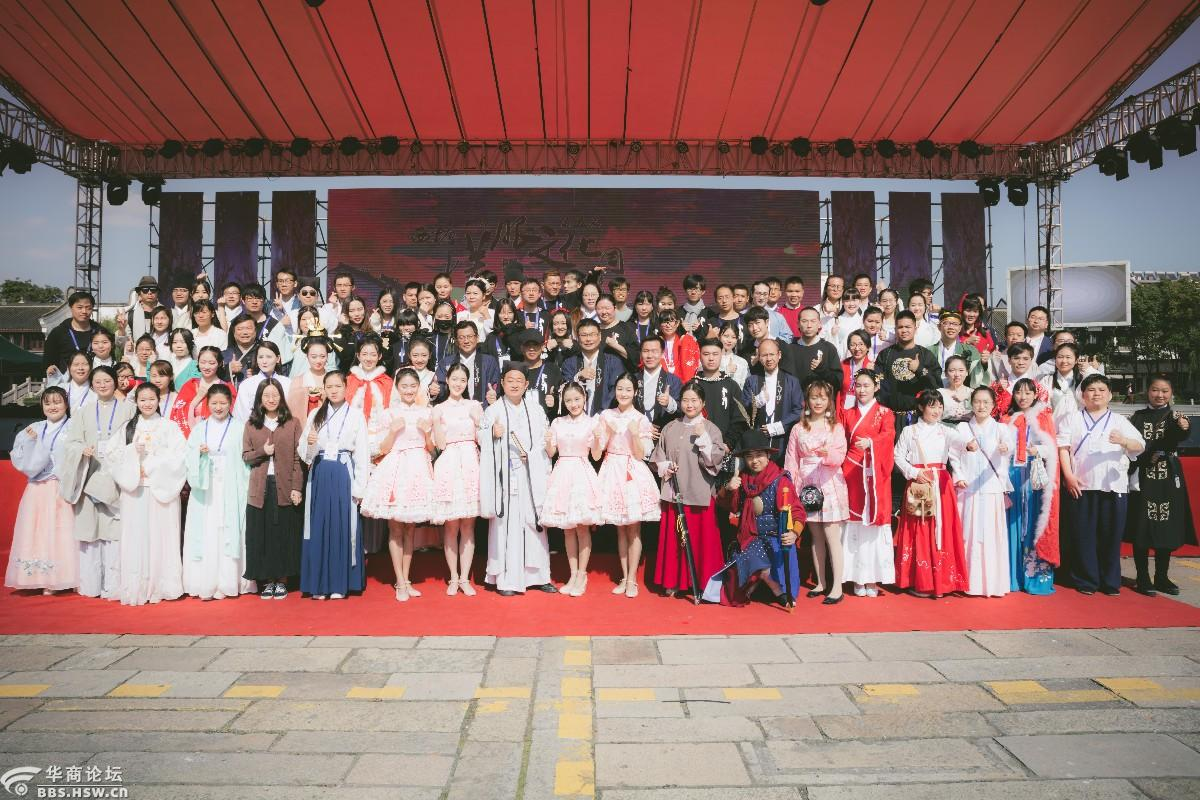•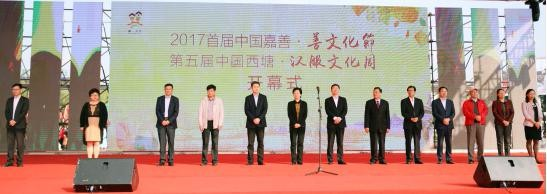•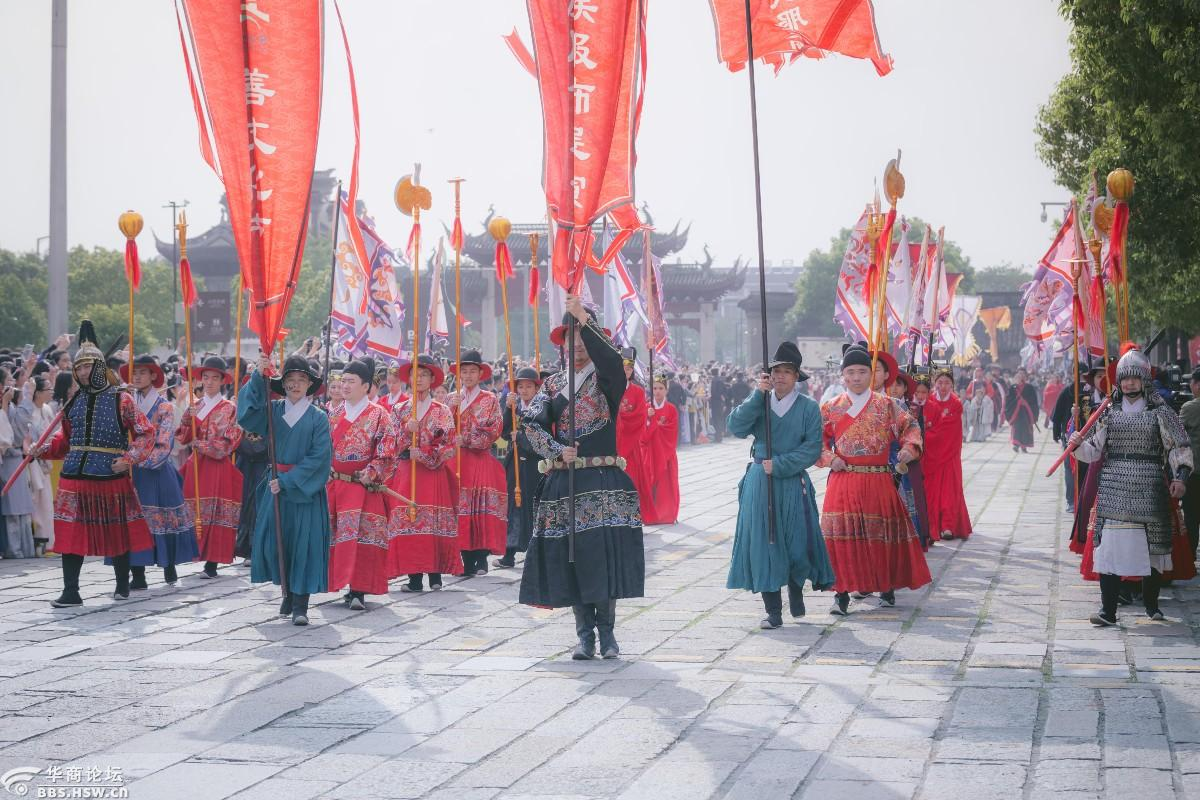限100 字节 如果您在写长篇帖子又不马上发表，建议存为草稿。 您目前还是游客，请 登录 或 注册 请广大网友文明发言，理性评论!    回复后跳转到最后一页
• ### 华商论坛 如何联系我们

（工作时间：7x24小时）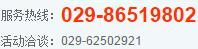全民乱拍

亲子活动

户外活动

华商车友会

无图版访问

手机版访问

触屏版访问

积分管理

管理条例

关注我们

• ### 华商论坛公众微信• ### 华商头条下载